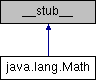FemtoIDE Java API  v0.1.2java.lang.Math Class Reference
Inheritance diagram for java.lang.Math:## Static Public Member Functions

static float toRadians (float deg)
Converts an angle measured in degrees to an approximately equivalent angle measured in radians. More...

static float toDegrees (float radians)
Converts an angle measured in radians to an approximately equivalent angle measured in degrees. More...

static double max (double a, double b)
Returns the greater of two `double` values.

static int max (int a, int b)
Returns the greater of two `int` values.

static float max (float a, float b)
Returns the greater of two `float` values.

static long max (long a, long b)
Returns the greater of two `long` values.

static uint max (uint a, uint b)
Returns the greater of two `uint` values. More...

static byte max (byte a, byte b)
Returns the greater of two `byte` values. More...

static double min (double a, double b)
Returns the lesser of two `double` values.

static int min (int a, int b)
Returns the lesser of two `int` values.

static float min (float a, float b)
Returns the lesser of two `float` values.

static long min (long a, long b)
Returns the lesser of two `long` values.

static uint min (uint a, uint b)
Returns the lesser of two `uint` values. More...

static byte min (byte a, byte b)
Returns the lesser of two `byte` values. More...

static double abs (double a)
Returns the absolute value of a `double` value.

static float abs (float a)
Returns the absolute value of a `float` value.

static int abs (int a)
Returns the absolute value of an `int` value.

static long abs (long a)
Returns the absolute value of a `long` value.

static float floor (float a)
Returns the largest (closest to positive infinity) `float` value that is less than or equal to the argument and is equal to a mathematical integer.

static float round (float a)

static float ceil (float a)

static float random ()
Returns a `float` value with a positive sign, greater than or equal to `0.0` and less than `1.0`.

static int random (int min, int max)
Returns an `int` value, greater than or equal to `min` and less than `max`

static float cos (float angle)
Returns the trigonometric cosine of an angle. The angle must be expressed in radians.

static float sin (float angle)
Returns the trigonometric sine of an angle. The angle must be expressed in radians.

static float atan2 (float fy, float fx)
Returns the angle theta from the conversion of rectangular coordinates (x, y) to polar coordinates (r, theta). More...

static float sqrt (float x)
Returns the square root of a `float` value.

static float signum (float f)
Returns the signum function of the argument; zero if the argument is zero, 1.0f if the argument is greater than zero, -1.0f if the argument is less than zero.

## Static Public Attributes

static final float PI = 3.1415926535897932384626433832795028841971f
The `float` value that is closer than any other to pi, the ratio of the circumference of a circle to its diameter.

static final float E = 2.7182818284590452353602874713526624977572f
The `float` value that is closer than any other to e, the base of the natural logarithms.

## ◆ atan2()

 static float java.lang.Math.atan2 ( float fy, float fx )
static

Returns the angle theta from the conversion of rectangular coordinates (x, y) to polar coordinates (r, theta).

The returned value is the arctangent of the quotient of its arguments, as a numeric value between `-PI` and `PI` radians. The number returned represents the anticlockwise/counterclockwise angle in radians between the positive x axis and the point (x, y).

Returns
An angle expressed in radians, between the values of `-PI` and `PI`.

## ◆ max() [1/2]

 static byte java.lang.Math.max ( byte a, byte b )
static

Returns the greater of two `byte` values.

Note
This function is non-standard

## ◆ max() [2/2]

 static uint java.lang.Math.max ( uint a, uint b )
static

Returns the greater of two `uint` values.

Note
This function is non-standard

## ◆ min() [1/2]

 static byte java.lang.Math.min ( byte a, byte b )
static

Returns the lesser of two `byte` values.

Note
This function is non-standard

## ◆ min() [2/2]

 static uint java.lang.Math.min ( uint a, uint b )
static

Returns the lesser of two `uint` values.

Note
This function is non-standard

## ◆ toDegrees()

 static float java.lang.Math.toDegrees ( float radians )
static

Converts an angle measured in radians to an approximately equivalent angle measured in degrees.

Parameters
Returns
An angle expressed in degrees.